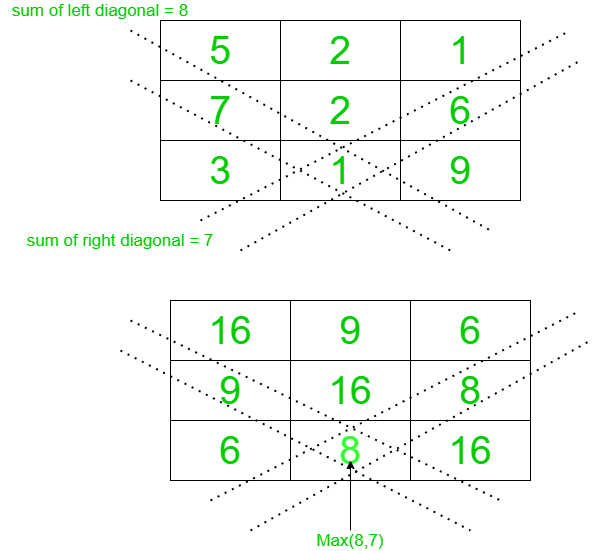Related Articles
Modify a matrix by replacing each element with the maximum of its left or right diagonal sum
• Last Updated : 10 Mar, 2021

Given a matrix mat[][] with dimensions M * N, the task is to replace each matrix elements with the maximum sum of its left or right diagonal.

Examples:

Input: mat[][] = {{5, 2, 1}, {7, 2, 6}, {3, 1, 9}}
Output:
16  9   6
9 16   8
6   8 16
Explanation:
Replace each element with max(sum of right diagonal, sum of left diagonal).
Follow the diagram below to understand more clearly.Input: mat[][] = {{1, 2}, {3, 4}}
Output:
5 5
5 5

Approach: The main idea is based on the facts based on the observation stated below:

• Sum of row and column indices for the right diagonal elements are equal.
• Difference between the row and column indices for left diagonal elements is equal.
• Using the above two properties to store the sum of the left and right diagonals of each element using a Map.
• Traverse the matrix and replace each element with the maximum of left diagonal sum or right diagonal sum.
• Print the final matrix obtained.

Below is the implementation of the above approach:

## C++

 `// C++ program for the above approach``#include ``using` `namespace` `std;` `// Function to update given matrix with``// maximum of left and right diagonal sum``void` `updateMatrix(``int` `mat[])``{` `  ``// Stores the total sum``  ``// of right diagonal``  ``map<``int``, ``int``> right;` `  ``// Stores the total sum``  ``// of left diagonal``  ``map<``int``, ``int``> left;` `  ``for` `(``int` `i = 0; i < 3; i++) {``    ``for` `(``int` `j = 0; j < 3; j++) {` `      ``// Update the map storing``      ``// right diagonal sums``      ``if` `(right.find(i + j) == right.end())``        ``right[i + j] = mat[i][j];``      ``else``        ``right[i + j] += mat[i][j];` `      ``// Update the map storing``      ``// left diagonal sums``      ``if` `(left.find(i - j) == left.end())``        ``left[i - j] = mat[i][j];``      ``else``        ``left[i - j] += mat[i][j];``    ``}``  ``}``  ` `  ``// Traverse the matrix``  ``for` `(``int` `i = 0; i < 3; i++) {``    ``for` `(``int` `j = 0; j < 3; j++) {` `      ``// Update the matrix``      ``mat[i][j] = max(right[i + j], left[i - j]);``    ``}``  ``}` `  ``// Print the matrix``  ``for` `(``int` `i = 0; i < 3; i++) {``    ``for` `(``int` `j = 0; j < 3; j++) {``      ``cout << mat[i][j] << ``" "``;``    ``}``    ``cout << endl;``  ``}``}` `// Driver code``int` `main()``{``  ``int` `mat[]``    ``= { { 5, 2, 1 }, { 7, 2, 6 }, { 3, 1, 9 } };``  ``updateMatrix(mat);``  ``return` `0;``}` `// This code is contributed by ukasp.`

## Python3

 `# Python3 program for the above approach` `# Function to update given matrix with``# maximum of left and right diagonal sum``def` `updateMatrix(mat):` `    ``# Stores the total sum``    ``# of right diagonal``    ``right ``=` `{}` `    ``# Stores the total sum``    ``# of left diagonal``    ``left ``=` `{}` `    ``for` `i ``in` `range``(``len``(mat)):``        ``for` `j ``in` `range``(``len``(mat[``0``])):` `            ``# Update the map storing``            ``# right diagonal sums``            ``if` `i ``+` `j ``not` `in` `right:``                ``right[i ``+` `j] ``=` `mat[i][j]``            ``else``:``                ``right[i ``+` `j] ``+``=` `mat[i][j]` `            ``# Update the map storing``            ``# left diagonal sums``            ``if` `i``-``j ``not` `in` `left:``                ``left[i``-``j] ``=` `mat[i][j]``            ``else``:``                ``left[i``-``j] ``+``=` `mat[i][j]` `    ``# Traverse the matrix``    ``for` `i ``in` `range``(``len``(mat)):``        ``for` `j ``in` `range``(``len``(mat[``0``])):` `            ``# Update the matrix``            ``mat[i][j] ``=` `max``(right[i ``+` `j], left[i``-``j])` `    ``# Print the matrix``    ``for` `i ``in` `mat:``        ``print``(``*``i)` `# Given matrix``mat ``=` `[[``5``, ``2``, ``1``], [``7``, ``2``, ``6``], [``3``, ``1``, ``9``]]``updateMatrix(mat)`
Output:
```16 9 6
9 16 8
6 8 16```

Time Complexity: O(N * M)
Auxiliary Space: O(N * M)

Attention reader! Don’t stop learning now. Get hold of all the important DSA concepts with the DSA Self Paced Course at a student-friendly price and become industry ready.

My Personal Notes arrow_drop_up